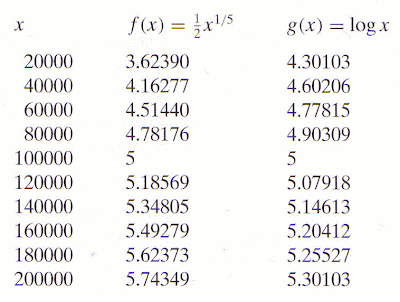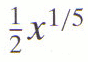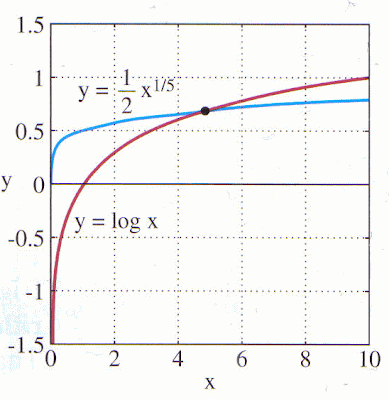## 25 Jul 2012

### The Nature of Logarithmic Functions. Logarithmic Functions in Edwards & Penney's Calculus

Edwards & Penney's Calculus is an incredibly-impressive, comprehensive, and understandable book. I highly recommend it.

[Search Blog Here. Index-tags are found on the bottom of the left column.]

[I your author am not a mathematician; I am merely an admirer of Edwards & Penney's wonderful calculus book. Please consult the text or other references to be certain about anything in the summary below. I mean this emphatically.]

The Nature of Logarithmic Functions
Logarithmic Functions in Edwards & Penney's Calculus

What do logarithmic functions got to do with you?

In the natural world around us, things often expand or tapper off in a steady way. Consider how sound reverberations taper off or how logarithmic spirals expand:

Logarithms can help us study many phenomena in the natural world around us.

Brief Summary

y = logax if ay = x

Points Relative to Deleuze

Deleuze writes:

Since intensity is already difference, it refers to a series of other differences that it affirms by affirming itself. It is said that in general there are no reports of null frequencies, no effectively null potentials, no absolutely null pressure, as though on a line with logarithmic gradations where zero lies at the end of an infinite series of smaller and smaller fractions. (Difference and Repetition 234c)

As a sound tapers off seemingly to nothing, it is perhaps undergoing an infinite series of lowering variations. The diminishing wave is not so much being negated as it is being constituted by infinitesimal differential relations.

Summary of
Edwards & Penney
Calculus

Chapter 1: Functions, Graphs, and Models
Section 1.4: Transcendental Functions

Subsection 4: Logarithmic Functions

Before we examine logarithmic functions, first recall exponential functions. In an exponential function, a constant base is raised to a variable power. A logarithmic function is an inverse to an exponential function. So consider this formulation for logarithmic functions:

y = logax if ay = x

Here we have a, y, and x. a is the base, y is an exponential power of a, and x is the value of a raised to the power of y. "The base a logarithm of the positive number x is the power to which a must be raised to get x." (p.39c)

Base 10 is the common logarithm: log10x

Later we discuss the natural logarithm e, which is a special irrational number.

lnx = logex

e = 2.71828182845904523536 . . . .

Edwarts and Penny graph y = lnx and y = log10x.Both graphs rise slowly and steadily as they move to the right, and also, both graphs cross through point (1, 0). Logarithmic functions cannot have negative numbers or zero in their domains, because exponential functions cannot take on zero or negative values. The function log x = log10x increases slowly as x increases. We see this in the graph and also in the fact that log10100,000 = 5 and log101,000,000 = 6. Consider how on the contrary in exponential function ax (with a > 1) growth increases more rapidly than any other powerfunction as x → ∞. The f0llowing example shows how logarithmic functions increase slower than power functions.

ExampleThis table compares the rate of growth in the power functionwith the rate of growth for the logarithmic function g(x) = logx. Here are graphs displaying these values.As we can see in both the table and the graph, when x > 100,000, logx is smaller thanThe graph below shows us the values when x is much smaller.Here we see that logx at the lower values begins smaller thanbut when x nears 5, the growth of logx overtakesThen much later in their development,overtakes logx when x = 100,000. Then, when x = 1050,equals 5,000,000,000, while logx only equals 50.

Deleuze, Gilles. Difference & Repetition. Transl. Paul Patton. New York: Columbia University Press, 1994.

Text summary and images from:
Edwards & Penney: Calculus. New Jersey: Prentice Hall, 2002, pp.39-40.

Shell image from:
http://en.wikipedia.org/wiki/File:NautilusCutawayLogarithmicSpiral.jpg